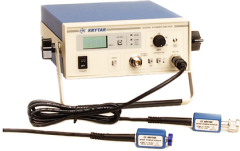# Power Meters InformationPower meters are used for high-accuracy measurements of power over a wide-frequency bandwidth, and from both AC and DC circuits. Measurements can be obtained for single-phase and three-phase AC systems. Some power meters can measure true RMS power. Root mean square (RMS) is the value obtained when the maximum voltage or current is divided by the square root of two. Power meters can be used for the measurement of power in various conditions, including half-wave and full-wave rectified waveforms, mixed AC/DC waveforms, and distorted waveforms.

## Performance Specifications

Performance specifications for power meters include:

• Power range
• Number of channel
• Sampling rate
• Type of power to measure

There are four measurement types:

• Active power
• Apparent power
• Reactive power
• Power factor

Active power is the time rate of transferring or transforming energy. The electrical power at a single terminal-pair is the product of the voltage and current. Apparent power is the product of the RMS values of voltage and current. Reactive power is the product of the RMS value of the voltage and the RMS value of the quadrature component of the current. Finally, power factor or quality factor is the ratio of active power to apparent power.

Phase angle parameters are also important product specifications for power meters. The phase angle is the angle, in degrees, between the current waveform and the voltage waveform. A leading phase angle means that the current is ahead of the voltage and the load is capacitive. If the current lags the voltage, then the phase angle is lagging and the load is inductive.

## Features

Power meters are available with a variety of features.

• External shunts can be used to extend the current input range.
• Integrating functions allow the active power and current to be integrated (Wh and Ah).
• Scaling functions allow the potential transformer and current transformer ratio to be preset.
• Automatic range switching means that when the measured value falls out of the rated range, the meter switches automatically to the appropriate unit for the range being measured.
• Harmonic analysis is phase measurement between three-phase inputs and measurement of active, reactive or apparent power of the fundamental wave.

Power meters can also support a wide range of current sensors used for evaluating inverter driven equipment and high frequency lighting equipment. Other, more general features for power meters include filtering, data logging, event triggering, and application software.

#### Image credit:

Krytar, Inc.